# Beginner's Guide to Fibonacci Forex Trading Strategy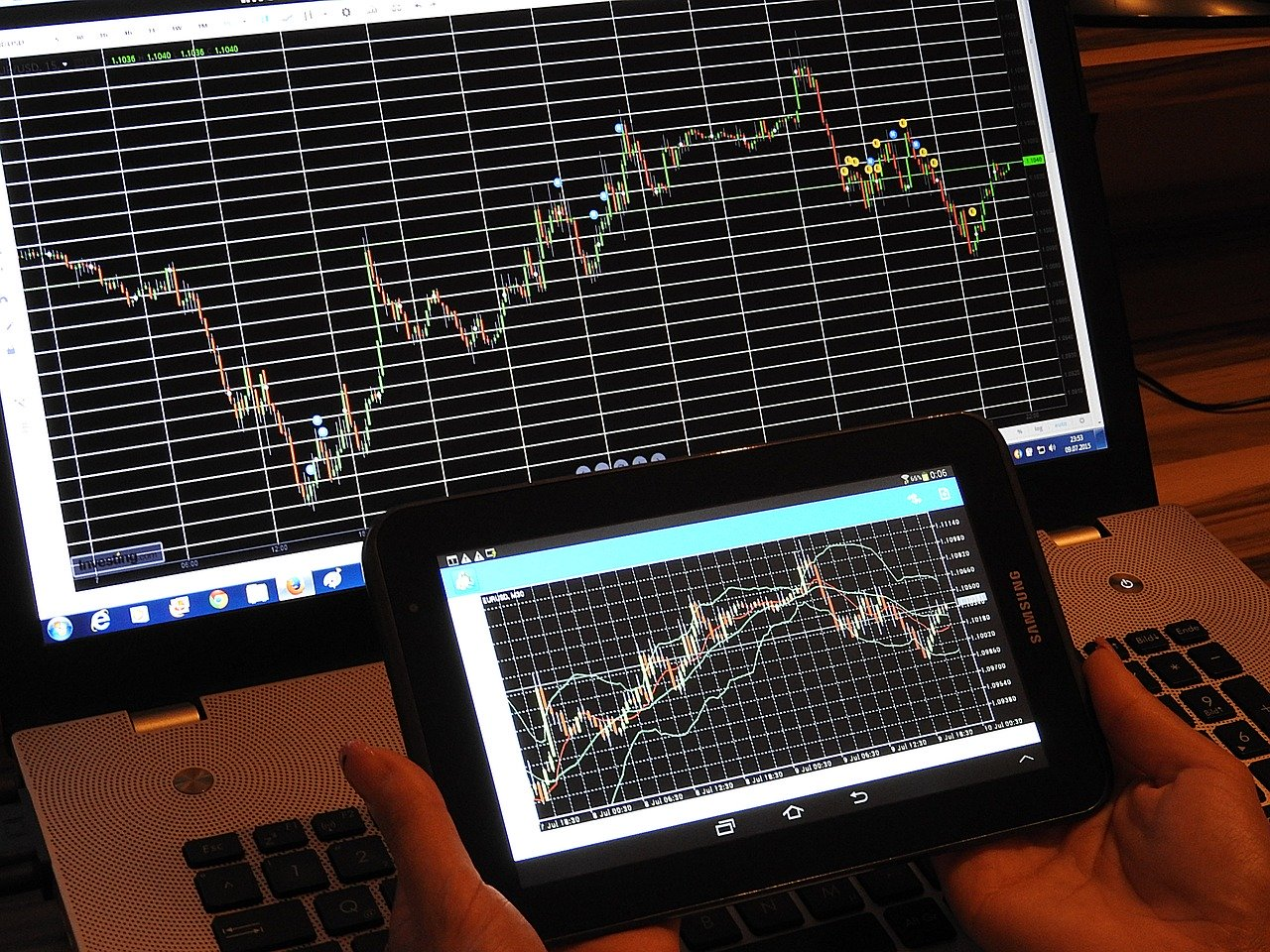Do you know Fibonacci is everywhere, even in the financial market? How can you increase the accuracy of your forex market analysis using a Fibonacci trading strategy? In the beginners' guide to Fibonacci, we will see what Fibonacci is along with its use in the Forex market with who you can trade in the forex market using Fibonacci tools.

21 February, 2020 | AtoZ Markets – If you are a technical analyst or in the Forex market for a long time, you may have heard the name of Fibonacci tools. Fibonacci tools are free in your MT4 and MT5 trading platform, but there are many traders who ignore it.

You can use Fibonacci besides your trading strategy to increase accuracy, or you can use Fibonacci as a trading strategy. Why does Fibonacci matter? Fibonacci matters because there is a deep relation between Fibonacci and nature.

## What is Fibonacci?

The Fibonacci sequence represents a certain numerical pattern that most of the high school algebra text covers. The sequence of numbers starts from zero and one, and then the next number comes with the addition of the previous two numbers. For example, the beginning of the sequence is 0, 1, 1, 2, 3, 5, 8, 13, 21, 34, 55, 89,144, 233, 377, and so on.

Why do These Numbers Matter?

This number matters, because these patterns are seen everywhere in the universe. Design of the human body to design the universe, Fibonacci is everywhere.

The model describes an amazing variety of phenomena, in mathematics, science, art, and nature. The mathematical ideas of the Fibonacci sequence lead to the golden ratio, spirals, and curves, which have meaning, charm, and beauty. However, no one can really explain why they are seen in the world of art and nature.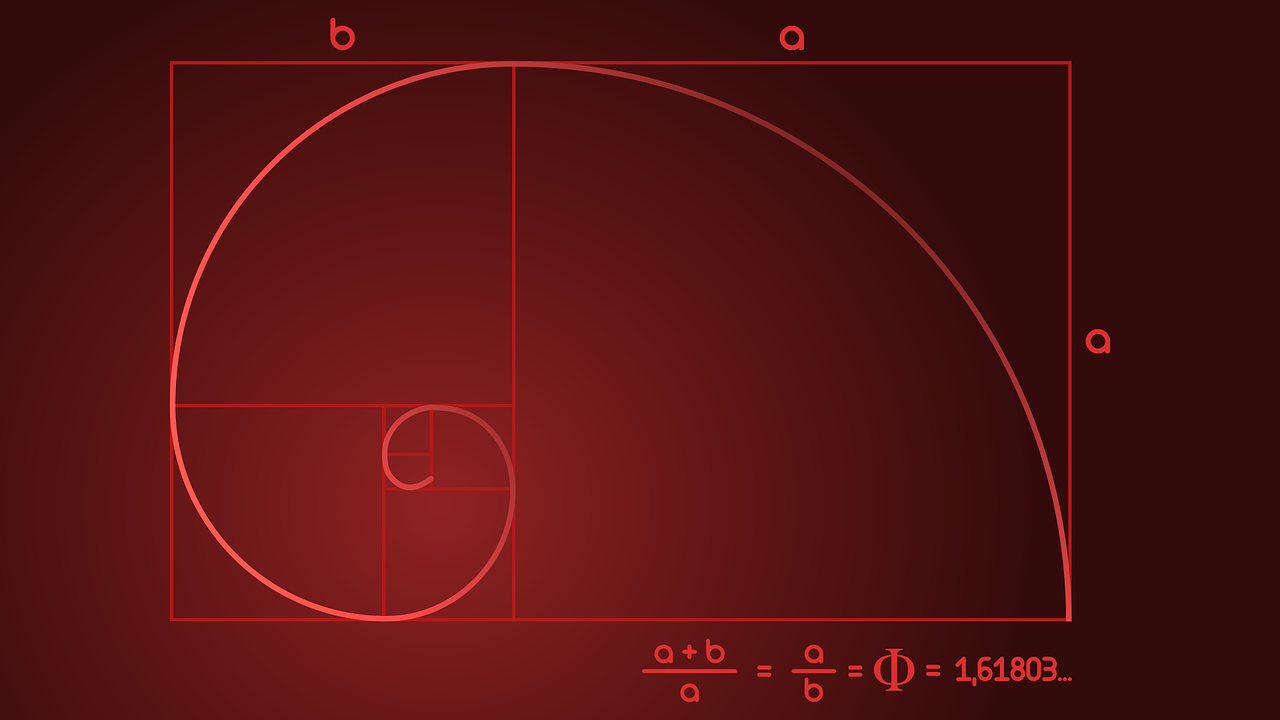For a forex trader, it is interesting to hear that the Fibonacci is seen in the financial market. Traders can predict the movement currency price, possible target area, and possible retracement area using Fibonacci tools.

Multibank Review
xm.com Review
XTB Review

## What is Fibonacci Trading Strategy?

Before proceeding to the forex market analysis using Fibonacci, let’s have a look at a bit details about the Fibonacci calculator. Later on, we will see why the calculation matters and how it is used in the forex market.

### Fibonacci Sequence in the Forex Market

The sequence of numbers starts from zero and one, and then the next number comes with the addition of the previous two numbers. For example, the beginning of the sequence is 0, 1, 1, 2, 3, 5, 8, 13, 21, 34, 55, 89,144, 233, 377, 610, 987, 1597, 2584, 4181, 6765….

There are some relations between these numbers with the forex charts to determine the possible price movement. Some basic calculations of Fibonacci are mentioned below-

• When you divide a number by the previous number, the result will be approximately 1.618. Traders use this number as a Fibonacci extension level.
• If you divide a number by the next number, the result may come as 0.618. Traders use this number as a 61.8% Fibonacci retracement level.
• When you divide a number by another two places higher, you will see the result to come as 0.382. This number represents the 38.2% Fibonacci retracement level.

1.618 is often known as the Golden Ratio, or Phi. The inverse of the golden ratio is 0.618. Therefore, both numbers are very significant in nature, biology, cosmos and in the forex market.

So, how can we use the Golden Ratio and other Fibonacci levels in forex trading? First, we need to split numbers into two different categories- Fibonacci retracement and Fibonacci extension. Both of these values will provide a possible turning point that could take place in the market.

### Use of Fibonacci Retracement Levels

Fibonacci retracement levels help to provide the price level from where a reversal may take place to establish entry levels. The retracement levels are based on two scenarios of the market:

• After completing a bullish trend, traders will point the Fibonacci indicators from bottom to top to find the possible retracement levels before bouncing higher.
• After completing a bearish trend, traders will point the Fibonacci indicators from top to bottom to find the possible retracement levels before bouncing lower.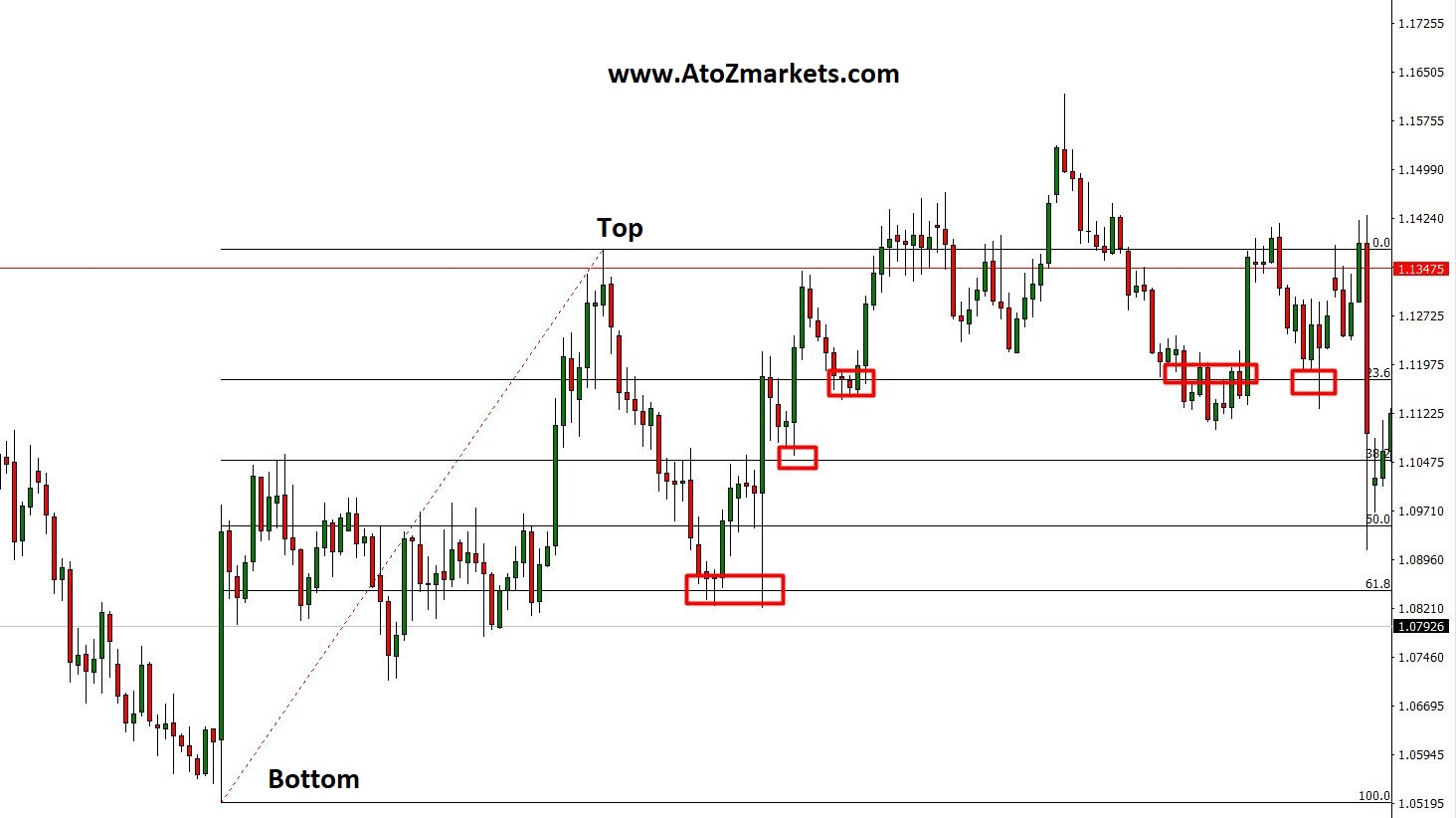If you look at the image, you may see how price reacted to the Fibonacci levels before moving further downside.

Conversely, price respected the Fibonacci levels to gain strengths before moving further upside.

Try the Fibonacci trading strategy on a free trading account with an AtoZ Approved broker:

Multibank Review
xm.com Review
XTB Review

### Use of Fibonacci Extension Levels

Fibonacci extension levels help to provide an indication of how far the price may go after getting a certain amount of retracement. Traders use the Fibonacci extension levels to determine the end of a trend.

As we discussed earlier, 1.618 is a key number in the Fibonacci sequence called the Golden Ratio. Therefore, the golden ratio is set in the price chart as a Fibonacci extension level of 161.8%.

• In an uptrend, traders will try to retrace the price at 61.8% before moving further high towards 161.8%.
• Similarly, in an uptrend, traders will try to retrace the price at 61.8% before moving further down towards 161.8%

Therefore, the Fibonacci retracement level helps to find possible support and resistance levels to enter a trade end. On the other hand, Fibonacci extension levels indicate how far the price may go before making any reversal.

The main and big reason to use the Fibonacci tools in the forex trading strategy is that- it works. As we know, Fibonacci is everywhere and there are many real examples of the golden ratio in nature. Therefore, traders believe that the 68.1% retracement and 161.8 extensions may give better accuracy compared to the other price prediction methods.

The forex market is run by big investors and central banks. Moreover, they spend a lot of money analyzing the market to get a better picture of what price can do next.

Therefore, big investors use Fibonacci tools due to its nature as a price predictor with better accuracy. Moreover, there are a lot of trading strategies with Fibonacci tools and there are many successful traders who often use Fibonacci in their trading strategies.

## Best MT4 and MT5 Fibonacci Trading Strategy

Here we will see a simple and powerful trading strategy using the Fibonacci tools that can be applied in both MT4 and MT5 trading platforms.

Besides the Fibonacci number, we will consider candlestick patterns to determine what buyers and sellers may react when the price approaches any significant level.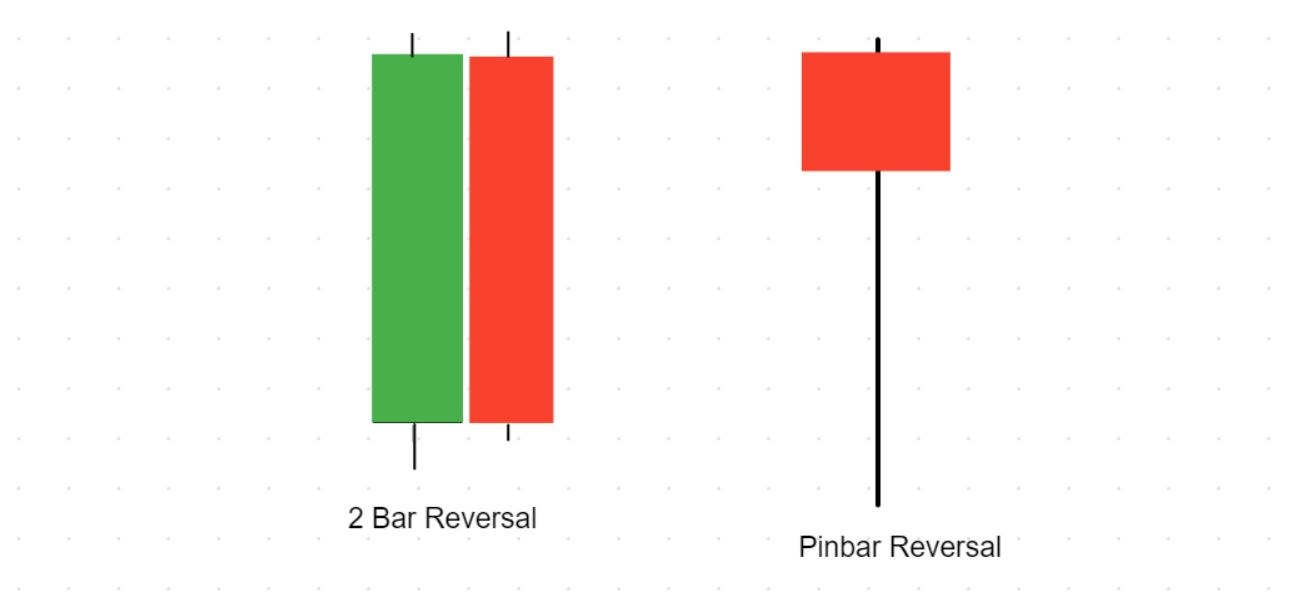In the above picture, we can see a pin bar and 2 bar candlestick pattern. These are the popular price action patterns that represent trend reversal. If the price moves higher and higher and suddenly a pin bar or 2 bar forms from a significant level, it is likely that the price will reverse. However, our main concern should be on what buyers and sellers may do when a pin bar or 2 bar forms by understanding the overall context using Fibonacci.

Like the Fibonacci trading strategy and want to try it out? Do it with an AtoZ Approved broker for free:

Multibank Review
xm.com Review
XTB Review

### Fibonacci Trading Strategy in an Uptrend

Let's start with a simple set of rules for when the market is in an uptrend:

• Identify the large uptrend (X to Y) and draw on Fibonacci retracement levels from the bottom to the top. The Fibonacci retracement tools are available free on MT4 and MT5 platforms.
• Identify price action trading patterns on a retracement level to determine what market movers are planning to do next.

After entering the trade, we need to set a possible target level for the trade-

Use the 161.8% Fibonacci extension level from the beginning to the end of the retracement.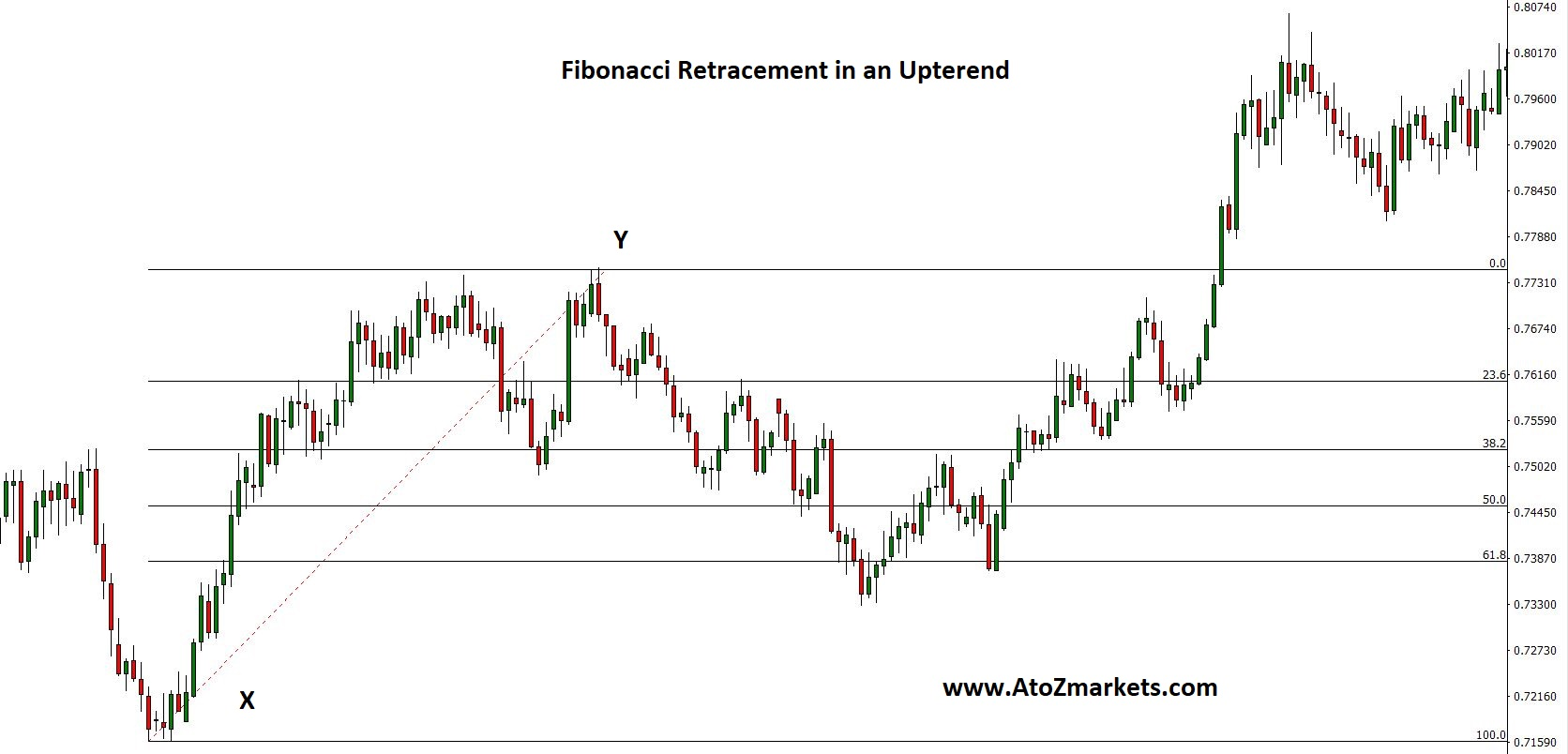In the example above, the price has retraced lower and formed a 2 bar price action pattern at the 61.8% Fibonacci retracement level. Moreover, it reached the 161.8% target level.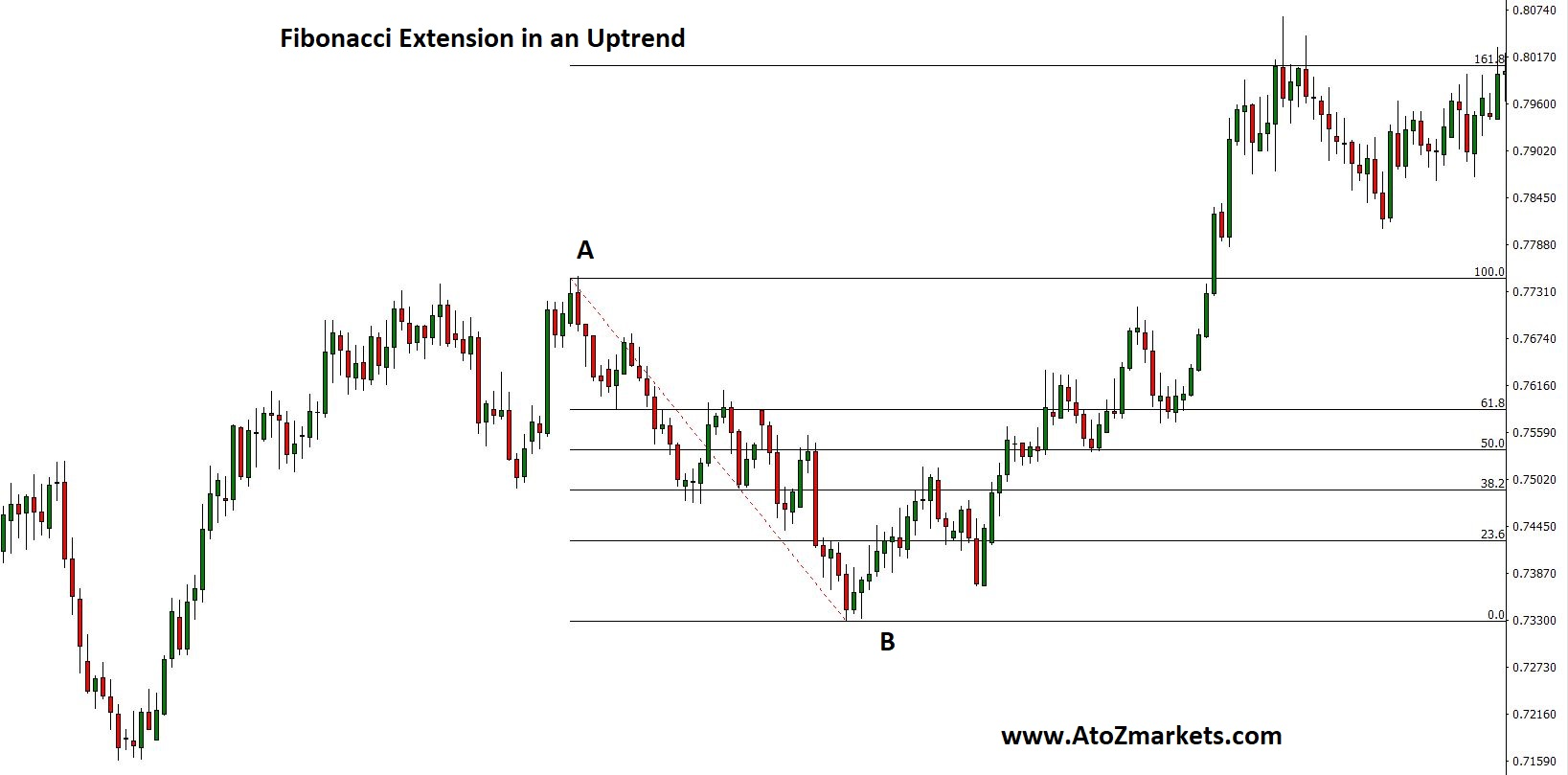In that case, buy entry is after closing the 2 bar pattern with stop loss below the pattern with some buffer. Therefore, the target would be the 161.8% extension.

### Fibonacci Trading Strategy on a Downtrend

Let's start with a simple set of rules for when the market is in a downtrend:

• Identify the large downtrend (X to Y) and draw on Fibonacci retracement levels from top to bottom.
• Identify price action trading patterns on a retracement level to determine what market movers are planning to do next.

After entering the trade, we need to set a possible target level for the trade-

Use the 161.8% Fibonacci extension level from the beginning to the end of the retracement.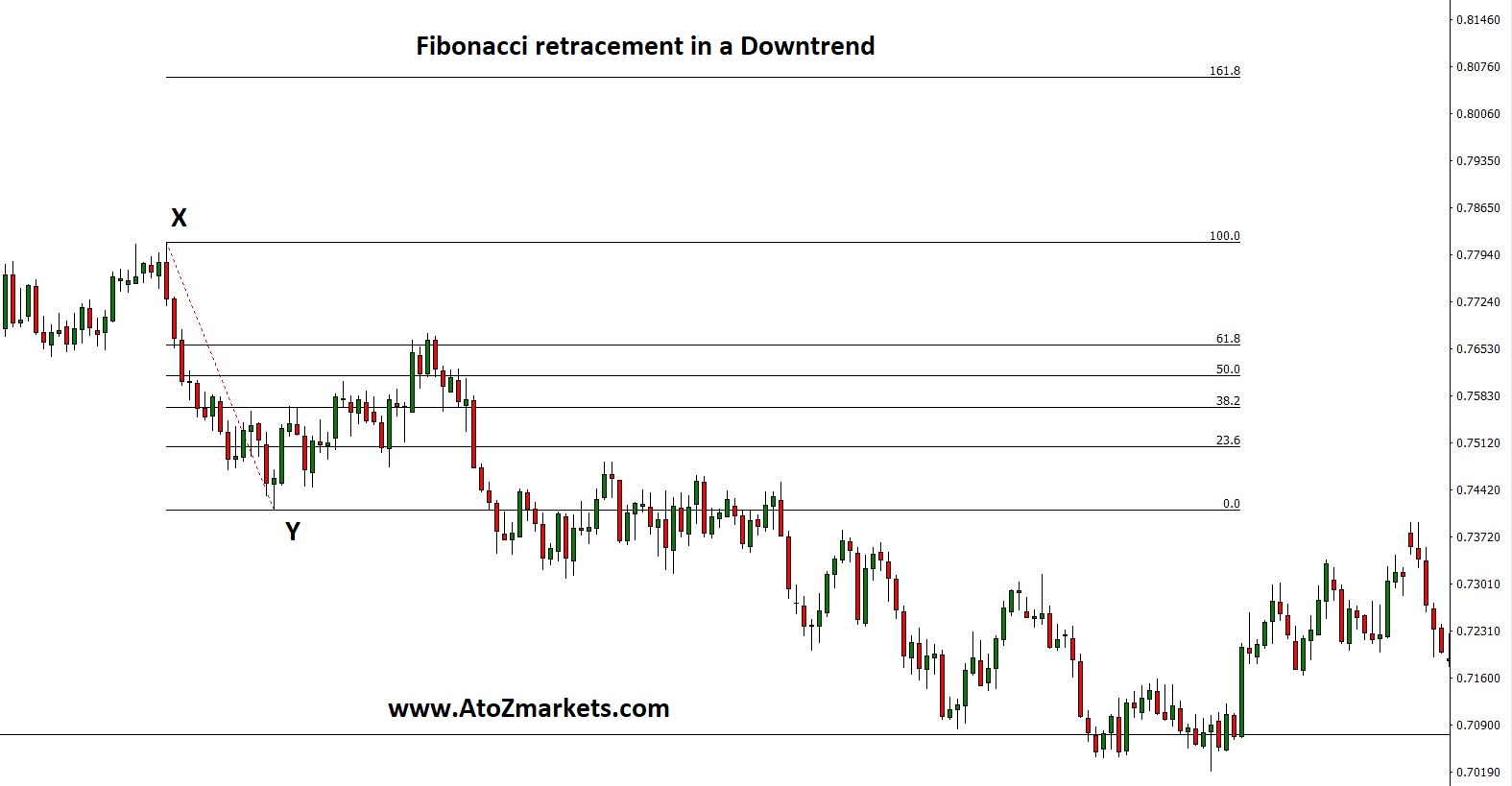In the example above, the price has retraced and formed a 2 bar price action pattern at the 61.8% Fibonacci retracement level. Moreover, it reached the 161.8% target level.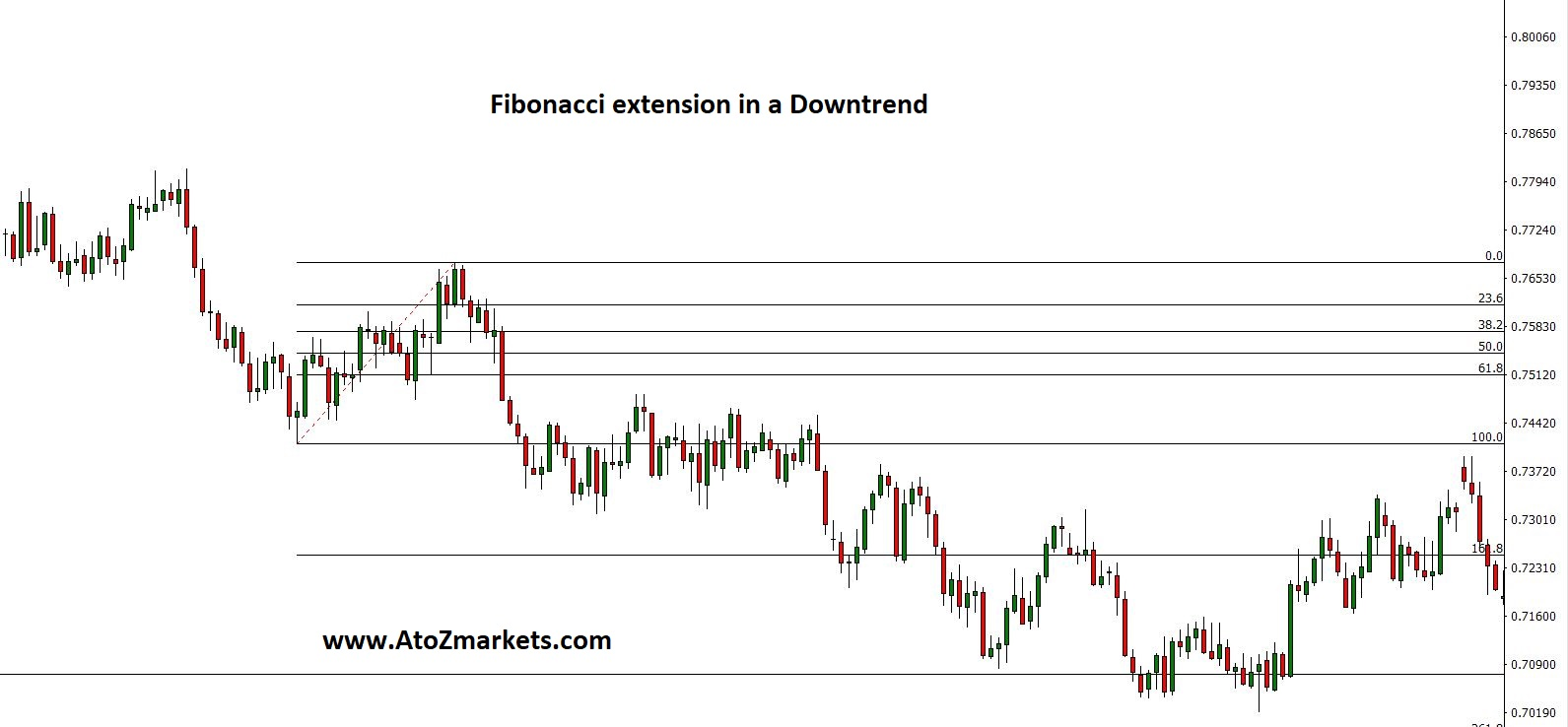In that case, you can take a sale entry after closing the 2 bar pattern with stop loss above the pattern with some buffer. Therefore, the target would be the 161.8% extension.

Multibank Review
xm.com Review
XTB Review

## Final Thoughts

The Fibonacci trading strategy is very popular and effective, whether it is a Forex market, Stock market or any other financial market.

Anyways, there are some risks associated with the Forex market. Therefore, no one can provide 100% accuracy in any market. Using Fibonacci can increase the probability of where the price can go in the coming days or periods.